Name:    3rd Grade Math Proficient Test 9

Multiple Choice
Identify the choice that best completes the statement or answers the question.

1.

Number Sense and Numeration – Whole Numbers – RIT 201 – 210

Round 236,562,547 to the nearest million
 a. 237,000,000 d. 236,600,000 b. 240,000,000 e. 23,000,000 c. 200,000,000

2.

If a four digit number is divisible by 2, 5, and 10, its last digit must be:
 a. 2 d. 4 b. 0 e. 9 c. 5

3.

The greatest common factor of 27 and 36 is:
 a. 3 d. 1 b. 9 e. 4 c. 6

4.

Number Sense and Numeration – Decimals – RIT 211 – 220

Round the following to the nearest cent: \$16.997
 a. \$16.99 d. \$16.00 b. \$16.10 e. \$20.00 c. \$17.00

5.

Which of the following fractions would become a repeating decimal?
 a. 1/8 d. 7/9 b. 3/4 e. 5/8 c. 27/36

6.

Number Sense and Numeration – Exponents – RIT 211 – 220

3,257 equals:
 a.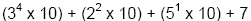d.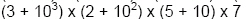b.e.c.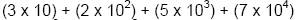7.

Express in scientific notation: 215,000,000
 a.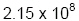d.b.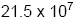e.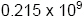c.8.

Express the following number in standard form: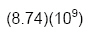a. 874,000 d. 8,740,000,000 b. 8,741,000,000 e. 874,000,000 c. 874,000,000,000

9.

Number Sense and Numeration – Fractions – RIT 211 – 220

Express the following as an improper fraction:a. 2 3/8 d. 19/24 b. 23/8 e. 5/8 c. 19/8

10.

What is the simplest form of 27/42?
 a. 27/42 d. 9/14 b. 1 15/27 e. 13/21 c. 2/3

11.

Number Sense and Numeration – Ordering, Equalities and Inequalities –
RIT 211 – 220

Order the following fractions from least to greatest:
4/8, 1/4, 4/6, 2/6
 a. 1/3, 2/3, 1/4, 2/4 d. 1/4, 2/6, 4/6, 4/8 b. 4/6, 4/8, 2/6, 1/4 e. 1/4, 2/6, 4/8, 4/6 c. 1/2, 1/3, 1/4, 2/3

12.

Which of the following is a true statement?
 a.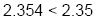d.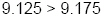b.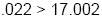e.c.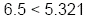13.

Number Sense and Numeration – Percents – RIT 211 – 220

Express 1/8 as both a decimal and a percent.
 a. 0.18 = 18% d. 0.125 = 12.5% b. 0.125 = 125% e. 0.13 = 13% c. 1.25 = 12.5%

14.

Number Sense and Numeration – Whole Numbers – RIT 211 – 220

Determine whether 42 is divisible by 2, 3, 5, 6, and/or 10.
 a. 2 d. 2, 3, 5, 6 b. 2, 3 e. 2, 3, 5, 10 c. 2, 3, 6

15.

Which are the greatest common factor and the least common multiple of the
numbers 12 and 9?
 a. G.C.F. = 3; L..C.M. = 24 d. G.C.F. = 1; L.C.M. = 108 b. G.C.F. = 3; L.C.M. = 36 e. G.C.F. = 3; L.C.M. = 27 c. G.C.F. = 6; L.C.M. = 108

16.

Problem Solving – RIT 191 – 200

What operation do the underlined words tell you to do?

Tom has 6 less CD’s than Marty.  What is the difference between the number of CD’s Tom and Marty have?
 a. add d. divide b. subtract e. use a fraction c. multiply

17.

If a movie ticket costs \$5.00, how much does it cost for 3 people to go to a
movie?
 a. \$15.00 d. \$2.00 b. \$12.00 e. \$23.00 c. \$8.00

18.

Estimate.
If a student is told to return to the library in about 1 hour, and it is 10:07 A.M.,
 a. 10:30 A.M. d. 11:00 P.M. b. 11:00 A.M. e. 10:00 P.M. c. 10:00 A.M.

19.

What operation should be used to solve the following question?
Ten students will play basketball. After school, they ask 5 more students to play
with them. How many students will play in all
 a. 15 d. multiplication b. subtraction e. division c. addition

20.

What problem solving strategy would best solve the following problem?
Sam has \$2.00 in his pocket when he got home. He spent \$2.00 at the candy
store, \$1.00 at the hobby shop, and found 50 on the way home. How much
money did Sam begin with?
 a. Guess and check d. Work backwards b. Look for a pattern e. Make a model c. Solve a simpler problem

21.

Joel needs 20 box tops to get a video game. He has 10 box tops and a
friend is giving him 7. How many more box tops will he need before he
can get the video game?
 a. 37 d. 10 b. 17 e. 3 c. 23

22.

Which number sentence will correctly solve this problem?
The children ate 8 slices of a pizza. The pizza was cut into 12 slices.
How many slices were left?
 a. 12 – 8 = 4 d. 20 – 8 = 12 b. 8 + 12 = 20 e. 20 – 4 = 16 c. 8 + 4 = 12

23.

Two brothers can share the money from selling the apples picked from
their grandmother’s apple tree. They pick 100 pounds of apples and sell
them for \$.50 a pound. How much money will each brother get?
 a. \$10.00 d. \$100.00 b. \$25.00 e. \$5.00 c. \$50.00

24.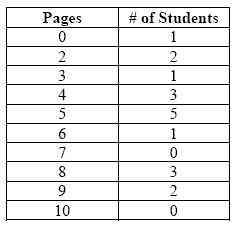The table shows the number of pages students read during silent reading
time. How many students read 5 or more pages?
 a. 17 d. 6 b. 5 e. 10 c. 11

25.

Which ratio represents the number of circles to squares?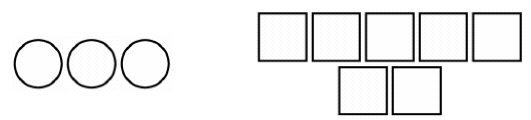a.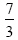d.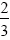b.e.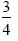c.26.

Trea has 11 sisters. They have all been asked to help their
Grandmother. Their Grandmother has a list of 6 things she needs the girls to
do. If each girl does her equal share, how many tasks will each girl do?
 a. 1 d. 6 b. 3 e. 2 c. 4